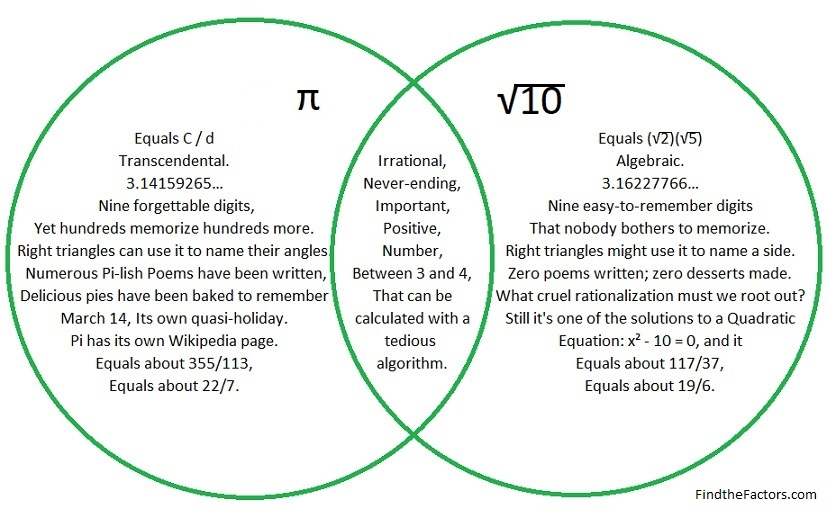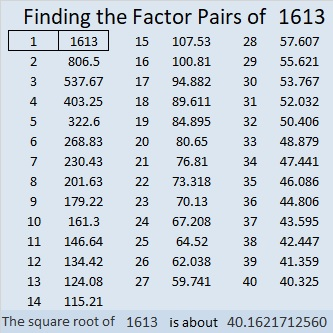# 1613 Comparing π and √10

Contents

### Venn Diagram Pies:

In the United States many people celebrate pi day. This year it will be one hour shorter as we move to Daylight Saving Time. Since it will be on a Sunday, it might not get as much attention in school. Do we make too much of a deal about the number pi? It’s about 0.02 less than √10, an important, yet less-known number. I compare the two numbers in this Venn diagram:We ought to take advantage of any reason to celebrate anything and everything in mathematics. I will be making some kind of pie to celebrate pi day, and I hope you do the same,

Now let’s move on to the ….

### Factors of 1613:

• 1613 is a prime number.
• Prime factorization: 1613 is prime.
• 1613 has no exponents greater than 1 in its prime factorization, so √1613 cannot be simplified.
• The exponent in the prime factorization is 1. Adding one to that exponent we get (1 + 1) = 2. Therefore 1613 has exactly 2 factors.
• The factors of 1613 are outlined with their factor pair partners in the graphic below.How do we know that 1613 is a prime number? If 1613 were not a prime number, then it would be divisible by at least one prime number less than or equal to √1613. Since 1613 cannot be divided evenly by 2, 3, 5, 7, 11, 13, 17, 19, 23, 29, 31, or 37, we know that 1613 is a prime number.

### More about the Number 1613:

1613 is the sum of two squares:
38² + 13² = 1613.

1613 is the hypotenuse of a Pythagorean triple:
988-1275-1613, calculated from 2(38)(13), 38² – 13², 38² + 13².

Here’s another way we know that 1613 is a prime number: Since its last two digits divided by 4 leave a remainder of 1, and 38² + 13² = 1613 with 38 and 13 having no common prime factors, 1613 will be prime unless it is divisible by a prime number Pythagorean triple hypotenuse less than or equal to √1613. Since 1613 is not divisible by 5, 13, 17, 29, or 37, we know that 1613 is a prime number.

## 2 thoughts on “1613 Comparing π and √10”

1.Steve Morris

So I guess Tuesday should be Root Ten Day!

•ivasallay

That could be fun. We could eat roots like potatoes, onions, carrots, parsnips, or beets. All of those appeal to me but probably not to kids…Lots of kids do like baby carrots, French fries, mashed potatoes and/or sweet potato pie, however!

This site uses Akismet to reduce spam. Learn how your comment data is processed.